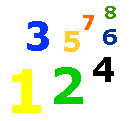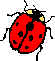# Problem Solving 1

Problem Solving Worksheet - My Schoolhouse - Online Learning

Erin has \$40.00.  Tickets for the basketball game cost \$9.00 each.   How many tickets can Erin buy?

tickets

Use the graph below.

 LADYBUGS COLLECTEDEachmeans 10 ladybugs. JillJohnTaraHow many ladybugs did each person collect?

Suppose each ladybug means 100 ladybugs.  How many did each person collect?

If 25 + 5 = 30, then 25 + 15 =

If 42 + 3 = 45, then 42 + 13 =

Count on and back by 100's.

 <-- COUNT BACK NUMBER COUNT ON --> 435 301 678 550